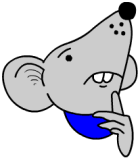# Free Y4 maths worksheet: Understand subtraction and its relationship to additionThis free maths worksheet looks at the relationship between addition and subtraction, in particular, that subtraction is the inverse or opposite of addition. This gives us an excellent way to check answers.

Look at 450 – 51.

We can do the subtraction sum and then check by doing the same subtraction sum again. But there is a danger with this that we might make the same mistake twice.

A better way is to do the subtraction and get the answer (399) and then check by adding the answer 399 to 51 which gives 450. Yes that is the same as the question.

Free Y 4 maths worksheet: Understand subtraction and its relationship to addition (pg 3)